# What is a GAN?

Let us take an analogy to explain the concept:

# How do GANs work?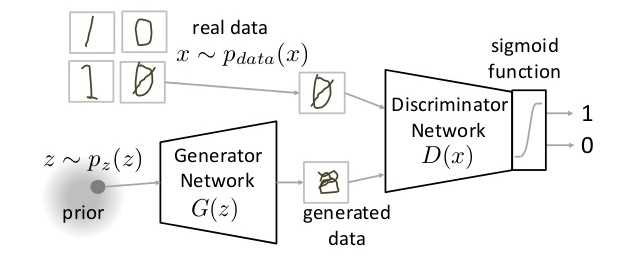The Generator Network($G(z)$) 接受随机噪声输入($z$ from $p(z)$)来产生假样本($g$). 随后送入 Discriminator Network $D(x)$. Discriminator Network 的任务是判断 real data 和 fake data 的真假. It takes an input $x$ from $P_{data}(x)$ where $P_{data}(x)$ is ourreal data distribution. $D(x)$ then solvers a binary classification problem using sigmoid function giving outpit in the range 0 to 1.

Now the training of GAN is done (as we saw above) as a fight between generator and discriminator. This can be represented mathematically as:

\begin{equation}
\label{a}
\mathop{min}\limits_{G} \mathop{max}\limits_{D}  V(D, G) \\
V(D, G) = E_{x \sim p_{data}(x)} [logD(x)] + E_{z \sim p_{z}(z)} [log(1-D(G(z))]
\end{equation}

train discriminator stage: 从判别器 $D$ 角度来看，它希望能尽可能区分出真假样本，即 maximize $V(D, G)$ to 0。具体来说，它一边希望 $D(x)$ 尽可能大，即 maximize $D(x)$ to 1. 另一边则希望 $D(G(z))$ 尽可能小，即 maximize $D(G(z))$ it to 0 (i.e. the log probability that the data from generated is fake is equal to 0).

train generator stage:  从生成器 $G$ 角度来看，它希望能够以假乱真 ，即 minimize the function $V$ to -NaN。 具体来说就是希望 $D(G(z))$ 尽可能大，即 maximize $D(G(x))$ to 1  (this stage only have second term).

Note: This method of training a GAN is taken from game theory called the minimax game.

# Parts of training GAN

So broadly a training phase has two main subparts and they are done sequentially:

• Pass 1: Train discriminator and freeze generator (freezing means setting training as false. The network does only forward pass and no backpropagationn is applied)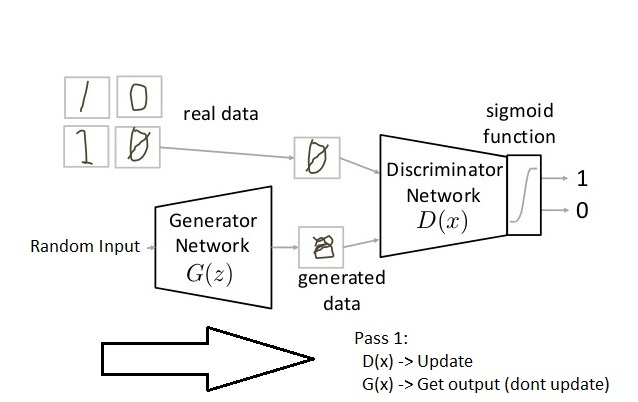• Pass 2: Train generator and freeze discriminator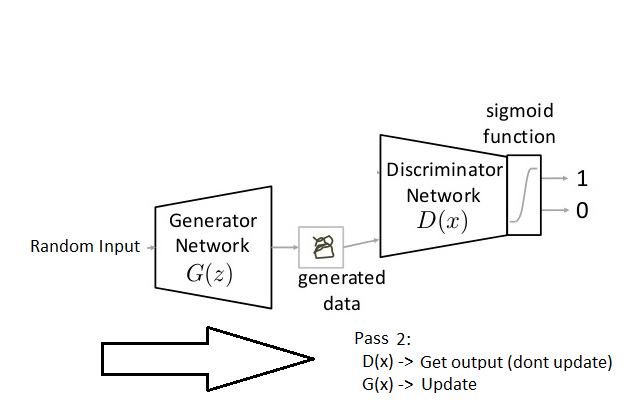# Steps to train a GAN

Step 1: Define the problem. Do you want to generate fake images or fake text. Here you should completely define the problem and collect data for it.

Step 2: Define architecture of GAN. Define how your GAN should look like. Should both your generator and discriminator be multi layer perceptrons, or convolutional neural networks? This step will depend on what problem you are trying to solve.

Step 3: Train Discriminator on real data for n epochs. Get the real data you want to generate fake on and train the discriminator to correctly predict them as real. Here value n can be any natural number between 1 and infinity.

Step 4: Generate fake inputs for generator and train Discriminator on fake data. Get generated data and let the discriminator correctly predict them as fake. (Step 3 and Step 4 are for Pass 1)

Step 5: Train Generator with the output of Discriminator. Now when the discriminator is trained, you can get its predictions and use it as an objective for training the generator. Train the generator to fool the discriminator. (This is Pass 2)

Step 6: Repeat step 3 to step 5 for a few epochs.

Step 7: Check if the fake data manually if it seems legit. If it seems appropriate, stop training, else go to step 3. This is a bit of a manual task, as hand evaluating the data is the best way to check the fakeness. When this step is over, you can evaluate whether the GAN is performing well enough.

# Challenges with GANs

There’s so many roadblocks into building a “good enough” GAN and we haven’t cleared many of them yet. There’s a whole area of research out there just to find “how to train a GAN

The most important roadblock while training a GAN is stability. If you start to train a GAN, and the discriminator part is much powerful that its generator counterpart, the generator would fail to train effectively. This will in turn affect training of your GAN. On the other hand, if the discriminator is too lenient; it would let literally any image be generated. And this will mean that your GAN is useless.

Another way to glance at stability of GAN is to look as a holistic convergence problem. Both generator and discriminator are fighting against each other to get one step ahead of the other. Also, they are dependent on each other for efficient training. If one of them fails, the whole system fails. So you have to make sure they don’t explode.

This is kind of like the shadow in Prince of Persia game . You have to defend yourself from the shadow, which tries to kill you. If you kill the shadow you die, but if you don’t do anything, you will definitely die!

There are other problems too, which I will list down here. (Reference: http://www.iangoodfellow.com/slides/2016-12-04-NIPS.pdf)

Note: Below mentioned images are generated by a GAN trained on ImageNet dataset.

• Problem with Counting: GANs fail to differentiate how many of a particular object should occur at a location. As we can see below, it gives more number of eyes in the head than naturally present.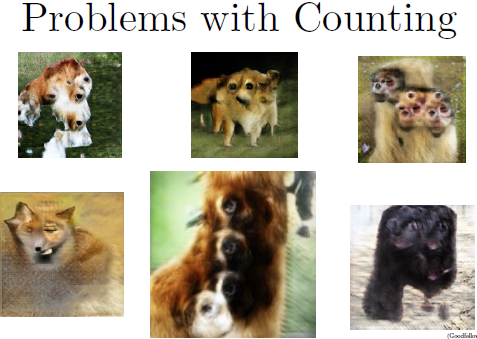• Problems with Perspective: GANs fail to adapt to 3D objects. It doesn’t understand perspective, i.e.difference between frontview and backview. As we can see below, it gives flat (2D) representation of 3D objects.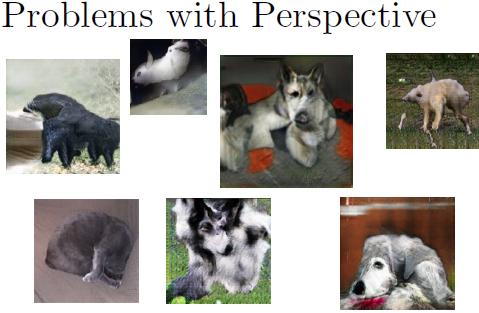• Problems with Global Structures: Same as the problem with perspective, GANs do not understand a holistic structure. For example, in the bottom left image, it gives a generated image of a quadruple cow, i.e. a cow standing on its hind legs and simultaneously on all four legs. That is definitely not possible in real life!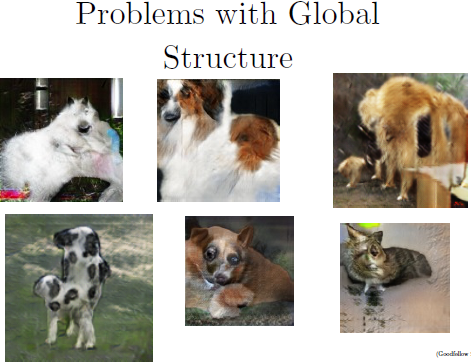A substantial research is being done to take care of these problems. Newer types of models are proposed which give more accurate results than previous techniques, such as DCGAN, WassersteinGAN etc

# Implementing a Toy GAN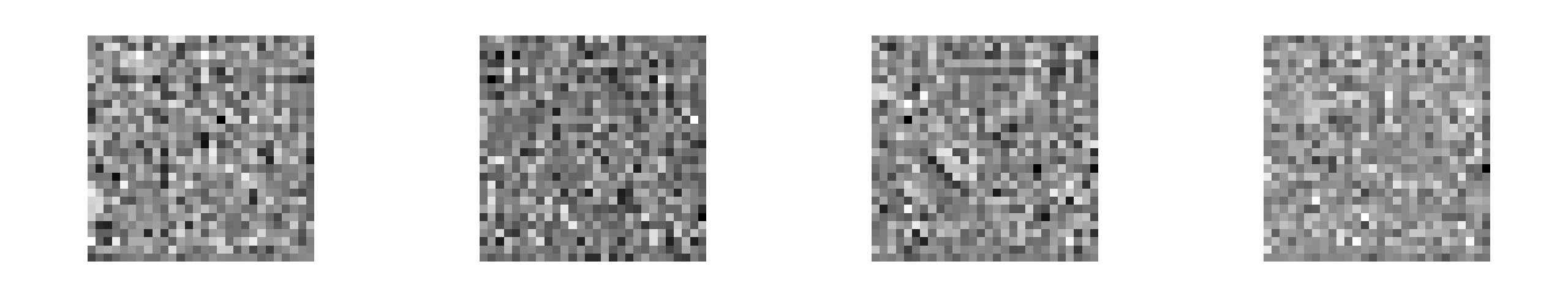## pytorch implementimport os
import torch
import torchvision
import torch.nn as nn
from torchvision import transforms
from torchvision.utils import save_image

# Device configuration
device = torch.device('cuda' if torch.cuda.is_available() else 'cpu')
print(torch.__version__, device)

# Hyper-parameters
latent_size = 64
hidden_size = 256
image_size = 784
num_epochs = 200
batch_size = 100
sample_dir = 'samples'

# Create a directory if not exists
if not os.path.exists(sample_dir):
os.makedirs(sample_dir)

# Image processing
transform = transforms.Compose([
transforms.ToTensor(),
transforms.Normalize(mean=(0.1307,),   # 3 for RGB channels
std=(0.3081,))])

# MNIST dataset
mnist = torchvision.datasets.MNIST(root='H:/Other_DataSets/MNIST/',
train=True,
transform=transform,

batch_size=batch_size,
shuffle=True)

# Discriminator
D = nn.Sequential(
nn.Linear(image_size, hidden_size),
nn.LeakyReLU(0.2),
nn.Linear(hidden_size, hidden_size),
nn.LeakyReLU(0.2),
nn.Linear(hidden_size, 1),
nn.Sigmoid())

# Generator
G = nn.Sequential(
nn.Linear(latent_size, hidden_size),
nn.ReLU(),
nn.Linear(hidden_size, hidden_size),
nn.ReLU(),
nn.Linear(hidden_size, image_size),
nn.Tanh())

# Device setting
D = D.to(device)
G = G.to(device)

# Binary cross entropy loss and optimizer
criterion = nn.BCELoss()

def denorm(x):
out = (x + 1) / 2
return out.clamp(0, 1)

# Start training
for epoch in range(num_epochs):
for i, (images, _) in enumerate(data_loader):
images = images.reshape(batch_size, -1).to(device)

# Create the labels which are later used as input for the BCE loss
real_labels = torch.ones(batch_size, 1).to(device)
fake_labels = torch.zeros(batch_size, 1).to(device)

# ================================================================== #
#                      Train the discriminator                       #
# ================================================================== #

# Compute BCE_Loss using real images where BCE_Loss(x, y): - y * log(D(x)) - (1-y) * log(1 - D(x))
# Second term of the loss is always zero since real_labels == 1
outputs = D(images)  # batch x 1
d_loss_real = criterion(outputs, real_labels)
real_score = outputs

# Compute BCELoss using fake images
# First term of the loss is always zero since fake_labels == 0
z = torch.randn(batch_size, latent_size).to(device)
fake_images = G(z) # batch x 784
outputs = D(fake_images) # batch x 1
d_loss_fake = criterion(outputs, fake_labels)
fake_score = outputs

# Backprop and optimize
d_loss = d_loss_real + d_loss_fake
d_loss.backward()
d_optimizer.step()

# ================================================================== #
#                        Train the generator                         #
# ================================================================== #

# Compute loss with fake images
z = torch.randn(batch_size, latent_size).to(device)
fake_images = G(z) # batch x 784
outputs = D(fake_images) # batch x 1

# We train G to maximize log(D(G(z)) instead of minimizing log(1-D(G(z)))
# For the reason, see the last paragraph of section 3. https://arxiv.org/pdf/1406.2661.pdf
g_loss = criterion(outputs, real_labels)

# Backprop and optimize
g_loss.backward()
g_optimizer.step()

if (i+1) % 200 == 0:
print('Epoch [{}/{}], Step [{}/{}], d_loss: {:.4f}, g_loss: {:.4f}, D(x): {:.2f}, D(G(z)): {:.2f}'
.format(epoch, num_epochs, i+1, total_step, d_loss.item(), g_loss.item(),
real_score.mean().item(), fake_score.mean().item()))

# Save real images
if (epoch+1) == 1:
images = images.reshape(images.size(0), 1, 28, 28)
save_image(denorm(images), os.path.join(sample_dir, 'real_images.png'))

# Save sampled images
fake_images = fake_images.reshape(fake_images.size(0), 1, 28, 28)
save_image(denorm(fake_images), os.path.join(sample_dir, 'fake_images-{}.png'.format(epoch+1)))

# Save the model checkpoints
torch.save(G.state_dict(), 'G.ckpt')
torch.save(D.state_dict(), 'D.ckpt')
View Code

## tensorflow implement#!/usr/bin/env python
# -*- coding: utf-8 -*-
# ================================================================
# @Time    : 2020/3/25 10:40
# @Author  : YangTao
# @Func    :
# @File    : GAN_example.py
# @IDE: PyCharm Community Edition
# ================================================================
import tensorflow as tf
from tensorflow.examples.tutorials.mnist import input_data
import numpy as np
import matplotlib.pyplot as plt
import matplotlib.gridspec as gridspec
import os

# 1. create data

def sample_Z(m, n):
return np.random.uniform(-1., 1., size=[m, n])

with tf.variable_scope('Input'):
X = tf.placeholder(dtype=tf.float32, shape=[None, 28 * 28], name='x')
Z = tf.placeholder(tf.float32, shape=[None, 100])

# 2. define Network
def xavier_init(size):
in_dim = size
xavier_stddev = 1. / tf.sqrt(in_dim / 2.)
return tf.random_normal(shape=size, stddev=xavier_stddev)

with tf.variable_scope('Net'):
D_W1 = tf.Variable(xavier_init([784, 128]))
D_b1 = tf.Variable(tf.zeros(shape=))

D_W2 = tf.Variable(xavier_init([128, 1]))
D_b2 = tf.Variable(tf.zeros(shape=))

theta_D = [D_W1, D_W2, D_b1, D_b2]

G_W1 = tf.Variable(xavier_init([100, 128]))
G_b1 = tf.Variable(tf.zeros(shape=))

G_W2 = tf.Variable(xavier_init([128, 784]))
G_b2 = tf.Variable(tf.zeros(shape=))

theta_G = [G_W1, G_W2, G_b1, G_b2]

def generator(z):
G_h1 = tf.nn.relu(tf.matmul(z, G_W1) + G_b1)
G_log_prob = tf.matmul(G_h1, G_W2) + G_b2
G_prob = tf.nn.sigmoid(G_log_prob)

return G_prob

def discriminator(x):
D_h1 = tf.nn.relu(tf.matmul(x, D_W1) + D_b1)
D_logit = tf.matmul(D_h1, D_W2) + D_b2
D_prob = tf.nn.sigmoid(D_logit)

return D_prob, D_logit

def plot(samples):
fig = plt.figure(figsize=(4, 4))
gs = gridspec.GridSpec(4, 4)
gs.update(wspace=0.05, hspace=0.05)

for i, sample in enumerate(samples):
ax = plt.subplot(gs[i])
plt.axis('off')
ax.set_xticklabels([])
ax.set_yticklabels([])
ax.set_aspect('equal')
plt.imshow(sample.reshape(28, 28), cmap='Greys_r')

return fig

G_sample = generator(Z)
D_real, D_logit_real = discriminator(X)
D_fake, D_logit_fake = discriminator(G_sample)

# D_loss = -tf.reduce_mean(tf.log(D_real) + tf.log(1. - D_fake))
# G_loss = -tf.reduce_mean(tf.log(D_fake))

# Alternative losses:
# -------------------
D_loss_real = tf.reduce_mean(
tf.nn.sigmoid_cross_entropy_with_logits(logits=D_logit_real, labels=tf.ones_like(D_logit_real)))
D_loss_fake = tf.reduce_mean(
tf.nn.sigmoid_cross_entropy_with_logits(logits=D_logit_fake, labels=tf.zeros_like(D_logit_fake)))
D_loss = D_loss_real + D_loss_fake

G_loss = tf.reduce_mean(tf.nn.sigmoid_cross_entropy_with_logits(logits=D_logit_fake, labels=tf.ones_like(D_logit_fake)))

mb_size = 128
Z_dim = 100

sess = tf.Session()
sess.run(tf.global_variables_initializer())

if not os.path.exists('out/'):
os.makedirs('out/')

i = 0

for it in range(1000000):
if it % 1000 == 0:
samples = sess.run(G_sample, feed_dict={Z: sample_Z(16, Z_dim)})

fig = plot(samples)
plt.savefig('out/{}.png'.format(str(i).zfill(3)), bbox_inches='tight')
i += 1
plt.close(fig)

X_mb, _ = mnist.train.next_batch(mb_size)

_, D_loss_curr = sess.run([D_solver, D_loss], feed_dict={X: X_mb, Z: sample_Z(mb_size, Z_dim)})
_, G_loss_curr = sess.run([G_solver, G_loss], feed_dict={Z: sample_Z(mb_size, Z_dim)})

if it % 1000 == 0:
print('Iter: {}'.format(it))
print('D loss: {:.4}'.format(D_loss_curr))
print('G_loss: {:.4}'.format(G_loss_curr))
print()
View Code

# Applications of GAN

We saw an overview of how these things work and got to know the challenges of training them. We will now see the cutting edge research that has been done using GANs

Increasing Resolution of an image

• Increasing Resolution of an image : Generate a high resolution photo from a comparatively low resolution.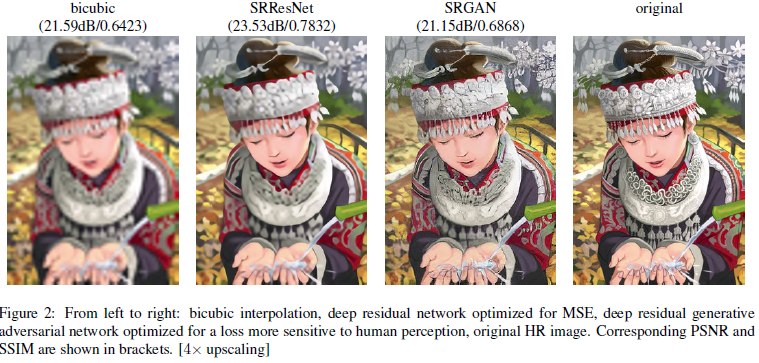Paper: https://arxiv.org/pdf/1609.04802.pdf
Code: https://github.com/tensorlayer/srgan
• Interactive Image Generation : Draw simple strokes and let the GAN draw an impressive picture for you!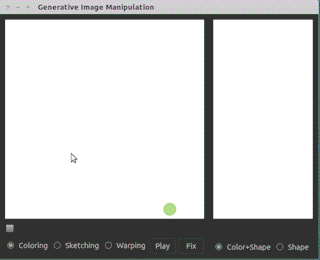• Image to Image Translation : Generate an image from another image. For example, given on the left, you have labels of a street scene and you can generate a real looking photo with GAN. On the right, you give a simple drawing of a handbag and you get a real looking drawing of a handbag.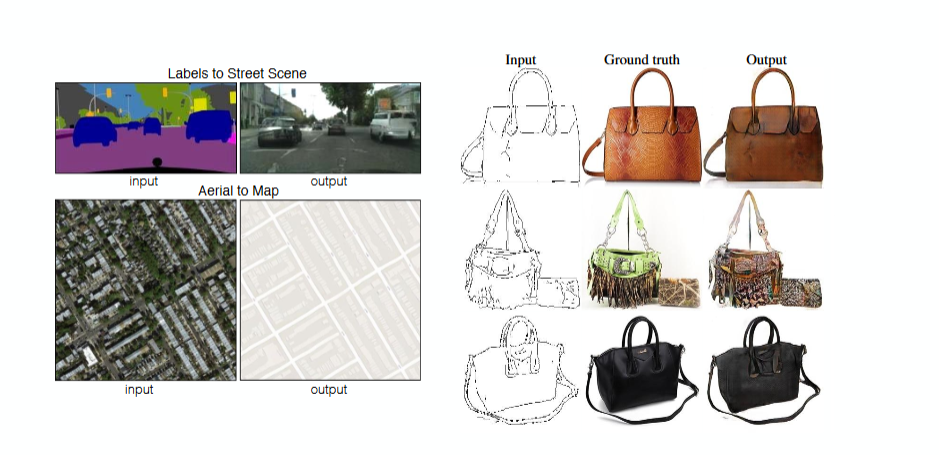Paper: https://arxiv.org/pdf/1611.07004.pdf

• Text to Image Generation : Just say to your GAN what you want to see and get a realistic photo of the target.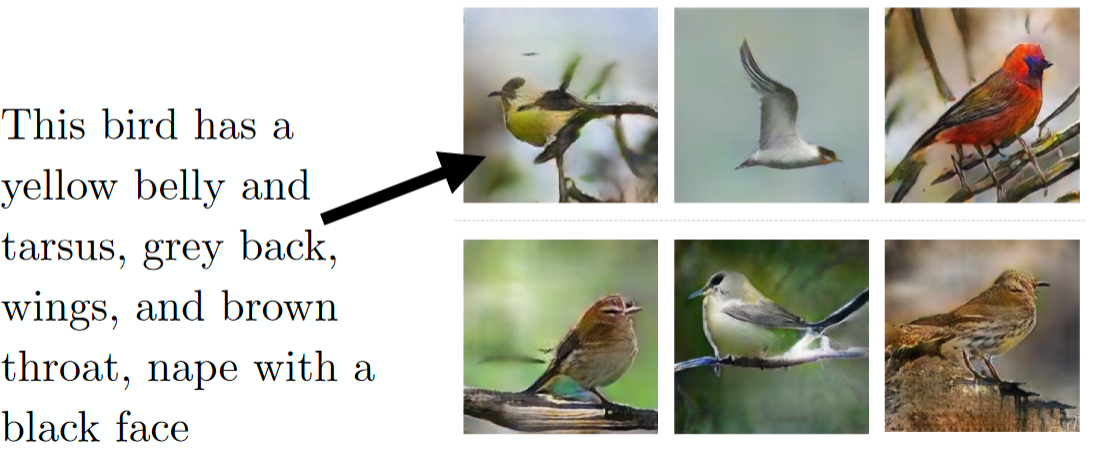Paper : https://arxiv.org/pdf/1605.05396.pdf

# Resources

Here are some resources which you might find helpful to get more in-depth on GAN

## End Notes

Phew! I hope you are now as excited about the future as I was when I first read about GANs. They are set to change what machines can do for us. Think of it – from preparing new recipes of food to creating drawings. The possibilities are endless.

In this article, I tried to cover a general overview of GAN and its applications. GAN is very exciting area and that’s why researchers are so excited about building generative models and you can see that new papers on GANs are coming out more frequently.

If you have any questions on GANs, please feel free to share them with me through comments.

# 其他链接

GAN论文阅读——原始GAN（基本概念及理论推导）

GAN 学习代码

GAN 论文合集

posted @ 2020-03-24 22:48  xuanyuyt  阅读(133)  评论(0编辑  收藏